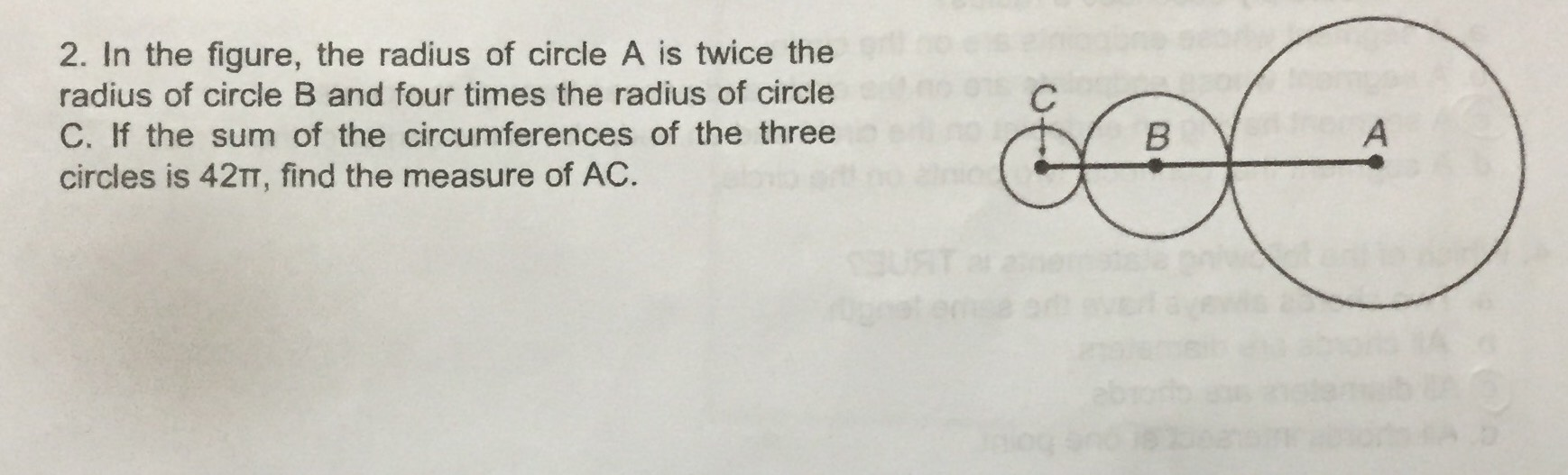Still have math questions?

Geometry
QuestionIn the figure, the radius of circle $$\mathrm{A}$$ is twice the radius of circle $$\mathrm{B}$$ and four times the radius of circle $$\mathrm{C}$$. If the sum of the circumferences of the three circles is $$42\pi$$, find the measure of $$\mathrm{AC}$$.

$$27$$